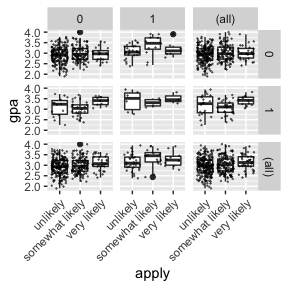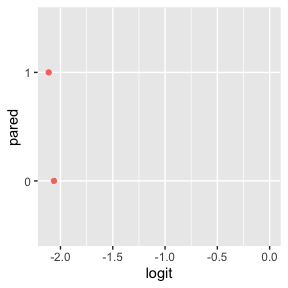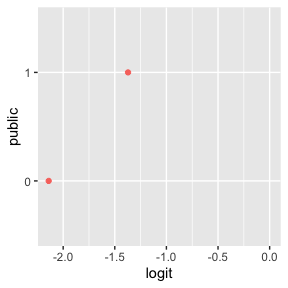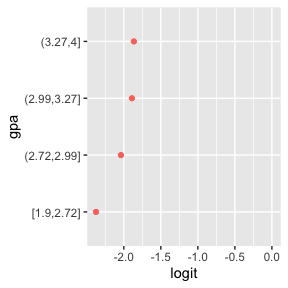# UCLA Statistical Consulting Example

This vignette replicates the ordinal logistic regression example done by the UCLA Statistical Consulting Group. It demonstrates how to use the pomcheckr package to check if the proportional odds assumption holds.

## Load packages and data

library(pomcheckr)
library(ggplot2)
data(ologit)

## Description of Data

head(ologit)
#> # A tibble: 6 x 4
#>   apply           pared public   gpa
#>   <fct>           <fct> <fct>  <dbl>
#> 1 very likely     0     0       3.26
#> 2 somewhat likely 1     0       3.21
#> 3 unlikely        1     1       3.94
#> 4 somewhat likely 0     0       2.81
#> 5 somewhat likely 0     0       2.53
#> 6 unlikely        0     1       2.59

ologit is a synthetic data set consisting of the following:

• apply - indicates how likely a student is to apply to graduate school

• pared - 1 if at least one parent has a graduate degree, 0 otherwise

• public - 1 if the undergraduate institution if public, 0 otherwise

• gpa - the student’s grade point average

## Descriptive Statistics

Some of the descriptive statistics from the example are repeated below.

## one at a time, table apply, pared, and public
lapply(ologit[, c("apply", "pared", "public")], table)
#> $apply #> #> unlikely somewhat likely very likely #> 220 140 40 #> #>$pared
#>
#>   0   1
#> 337  63
#>
#> \$public
#>
#>   0   1
#> 343  57
## three way cross tabs (xtabs) and flatten the table
ftable(xtabs(~ public + apply + pared, data = ologit))
#>                        pared   0   1
#> public apply
#> 0      unlikely              175  14
#>        somewhat likely        98  26
#>        very likely            20  10
#> 1      unlikely               25   6
#>        somewhat likely        12   4
#>        very likely             7   3
ggplot(ologit, aes(x = apply, y = gpa)) +
geom_boxplot() +
geom_jitter(size=0.1, alpha = .5) +
facet_grid(pared ~ public, margins = TRUE) +
theme(axis.text.x = element_text(angle = 45, hjust = 1, vjust = 1))## Analysis

The source page describes various analysis methods that one might consider and what the limitations are with respect to this data set. Since the outcome apply is an ordered, categorical variable an ordered logistic (aka cumulative logit) model is an appropriate choice.

### Proportional Odds Assumption

A key assumption of an ordinal logistic regression is that the odds of adjacent categories are proportional (i.e., the slope coefficients are the same). The score test is sometimes used to test this assumption, but it tends to be conservative and rejects the null more often than it should. The source page illustrates a graphical method for checking this assumption, and pomcheckr will automatically generate the necessary plots.

plot(pomcheck(apply ~ pared + public + gpa, data=ologit))The basic idea is a series of binary logistic regressions without the parallel slopes assumption are run on the response against the predictors. Then we check for equality of the slope coefficients across levels of the predictor (or cutpoints if the predictor is continuous). See the source page for further details.

In the above plots, the slope coefficients are roughly equal for both pared and gpa. However, the plot for public suggests the parallel slopes assumption is not satisfied for that predictor.